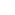Part of the Oxford Instruments Group
Expand

# How to Create Interactive Plots in Imaris Vantage | Tutorial

ImarisVantage is a statistical data-visualization module that allows you to understand complex statistical data by uncovering otherwise hidden relationships between objects and identifying patterns and trends.

ImarisVantage creates colorful high-dimensional plots in a way that makes the statistical data more meaningful. The plots are designed to enhance visual and intuitive understanding of the data by combining the numerical and graphical approaches. ImarisVantage lets you generate a hypothesis about the meaning of your data that can be easily tested with the appropriate Vantage plots.

To create a Vantage plot, you must first create a Surpass object. The object statistical data will be used for the plot creation. The statistical values of the object Spots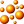, Surfaces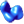, Cell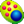, and Filaments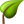could be used to create the Vantage plots.

To start ImarisVantage creation wizard follow these steps:

• Create and select the object in the Surpass tree
• In the Toolbars, click on the ImarisVantage icon
• ImarisVantage is instantly started and a list of all available objects for ImarisVantage plots is displayed.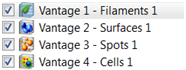The wizard shows a list of all available input components for ImarisVantage plots.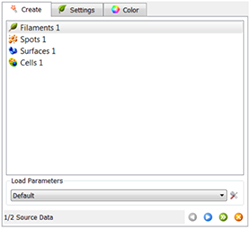Select one of the input components from the list (Spots, Surfaces, Cells, or Filaments). In the next step of the Creation Wizard, specify the plot type that you want to use. ImarisVantage lets you choose four different plot types.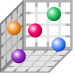XYZ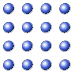Gallery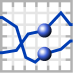Time Plot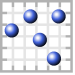Scatter Plot

Once you have determined what plot type you want to use, several predetermined subtypes are available based on the number of assigned plot dimensions. Under the Plot Values, all available plot dimensions are listed.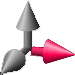XY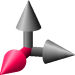Z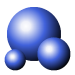Scale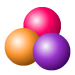Color

For each plot dimension, the drop-down list offers a set of available statistical variables for the selected object category.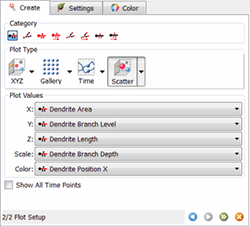To select the statistical variable to be assigned to the chosen plot dimension, click on its name and the selected variable will be automatically assigned.

Click on the Finish button and the Vantage plot is displayed in the viewing area. The plots characteristics are directly linked to the selected statistical variables. The collection of plots arrangements is limitless, letting you visualize, manage and analyze multi-dimensional data objects efficiently in one application.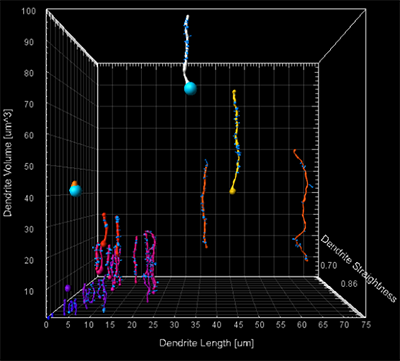Image above: Pyramidal Cell
Scatter plot
X plot dimension: Dendrite Length
Y plot dimension: Dendrite Volume
Z plot dimension: Dendrite Straightness
Color plot dimension: Dendrite Volume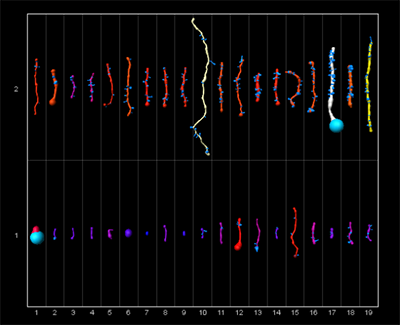Image above: Pyramidal Cell
Gallery plot
Sorted by: Dendrite No of Spines
Color plot dimension: Dendrite Area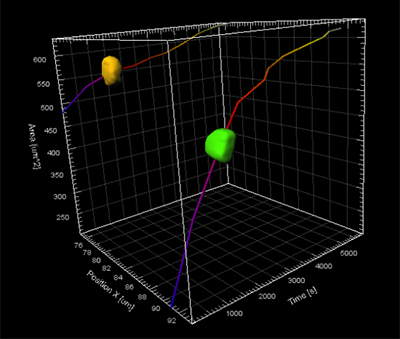Image above: HeLa Cell
Time plots
X plot dimension: Time
Y plot dimension: Area
Z plot dimension: Position X
Scale plot dimension: Speed
Color plot dimension: Sphericity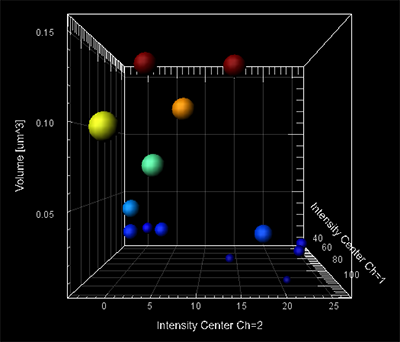Image above: PtK2Cell
Scatter plot
Intensity Center Ch2
Y plot dimension: Volume
Z plot dimension: Intensity Center Ch1
Color plot dimension: Volume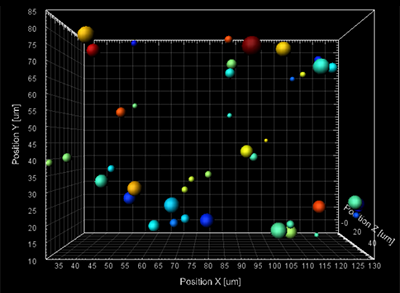Image above: Plant Cell
XYZ plots
X plot dimension: Position X
Y plot dimension: Position Y
Z plot dimension: Position Z
Scale plot dimension: Intensity Center Ch2
Color plot dimension: Area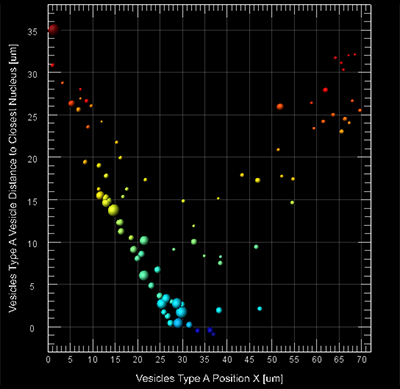Image above: Cell demo
Scatter plot
X plot dimension: Vesicle Type A Position X
Y plot dimension: Vesicle Type A Vesicle Distance to Closest Nucleus
Scale plot dimension: Vesicle Type A Intensity Max Ch3
Color plot dimension: Type A Vesicle Distance to Closest Nucleus

Date: December 2012

Category: How-to Guides